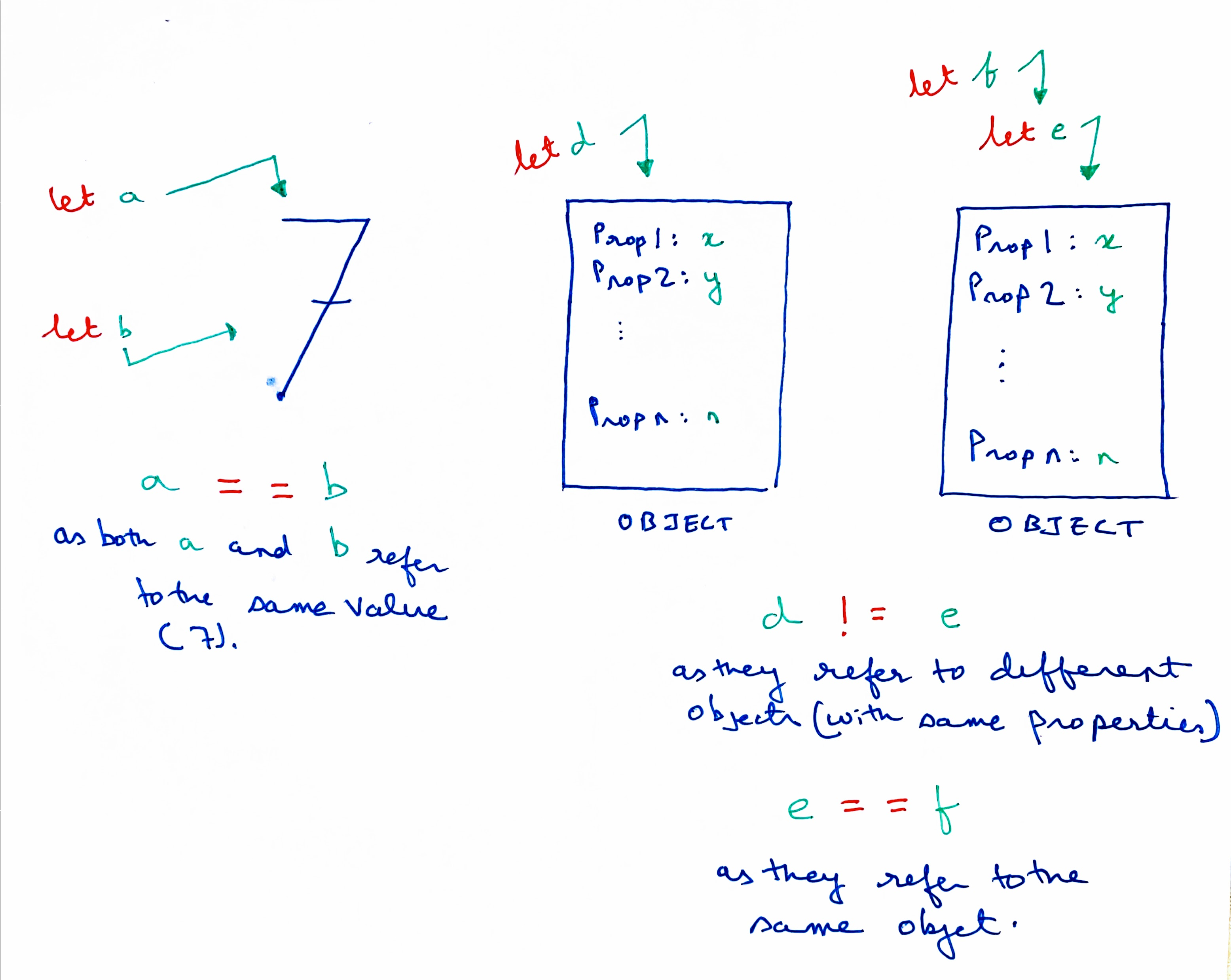# Data-types in Javascript

July 14, 2021

In this post, I try to understand the broad differences between primitive data-types and objects in Javascript.

## Types of values in Javascript

Data-types in Javascript can be classified into two buckets: primitives and objects.

There are six kinds of primitive data-types in Javascript, namely, `undefined`, `boolean`, `number`, `string`, `bigint`, and `symbol`

Objects, on the other hand, are unordered collections of properties (also referred to as complex primitives). Each object can have multiple properties. Each property has a name and a value. The names are typically strings that act as keys to their respective values. Effectively, an object is a collection of strings that act as identifiers to specific values.

An array is a special kind of object: they represent an ordered collection of sequential values. In a previous post, it was noted that, functions are treated like values in Javascript:

Effectively, functions are treated just as any other value is treated in Javascript. This is what makes functions first class citizens of Javascript.

In fact, functions are a considered as a special type of objects in `Javascript` .

## Differences between primitives and objects

### Immutability

Primitive values are, by definition, immutable. It is not possible to change the value of the number `9` or the boolean value `true`. Even strings are immutable in Javascript. This may seem counter-intuitive because it is possible to concatenate two strings. Similarly, it is possible to remove specific characters in a string

``````let str = "string";
str.substring(0,2);//this returns a new string: "str"
console.log(str);// output: "string"
str = str + "s"; //str now stores a new string: "strings"
``````

However, as shown above, the value of a `string` cannot be mutated; but a third string can created to contain the desired order of characters.

In contrast, objects are mutable data-types. The properties of an object can always be altered. (For this reason, it is permissible to add new properties or alter values of existing properties of objects defined by the keyword `const` , as seen in this post). As a corollary, there is no restriction to mutate the contents of an array either.

### Manner of comparison

Primitive values are always compared with respect to their respective values. This means that if the underlying values of two primitives match, they are considered to be same and indistinguishable. Thus, two strings would be considered equal to each other if they have the same number of characters and in the same sequence.

``````let str = "string";
let str2 = "string";
console.log(str === str2);//true
``````

But this is not the case for objects and arrays. Even if two objects contain the same number of properties, each of which have exactly the same names and store same values, Javascript will consider them as different objects. Same goes for arrays.

``````let obj = {};
let obj1 = {};
console.log(obj === obj1);//false
let arr = [1, 2, 3];
let arr1 = [1, 2, 3];
console.log(arr === arr1);//false
``````

Variables (and constants) are, in fact, identifiers to specific values. Thus, if two different variables are assigned the same primitive value, they both point to the same value. Hence, when they are compared, they are are found to be equal to each other.However, in the case of objects, when two variables are assigned two objects, which contain the same properties, each of these variables are, in fact, pointing to different objects, which happen to contain the same properties. However, if a variable is assigned another variable that points to an object, the two variables will be treated to be equal, because they are pointing to the same object. No new object was created for the second variable. Conversely, if the properties of the underlying object is modified, by using either of the variables, both the variables will reflect those changes. This is why objects are often referred to as reference types (as opposed to primitive types), as two object values will be considered to be same if and only if they both refer to the same object.

``````let obj = {};
let obj1 = obj;
obj1.name = "object";
console.log(obj);// { name: 'object' }
console.log(obj1);// { name: 'object' }
console.log(obj === obj1);//true
``````